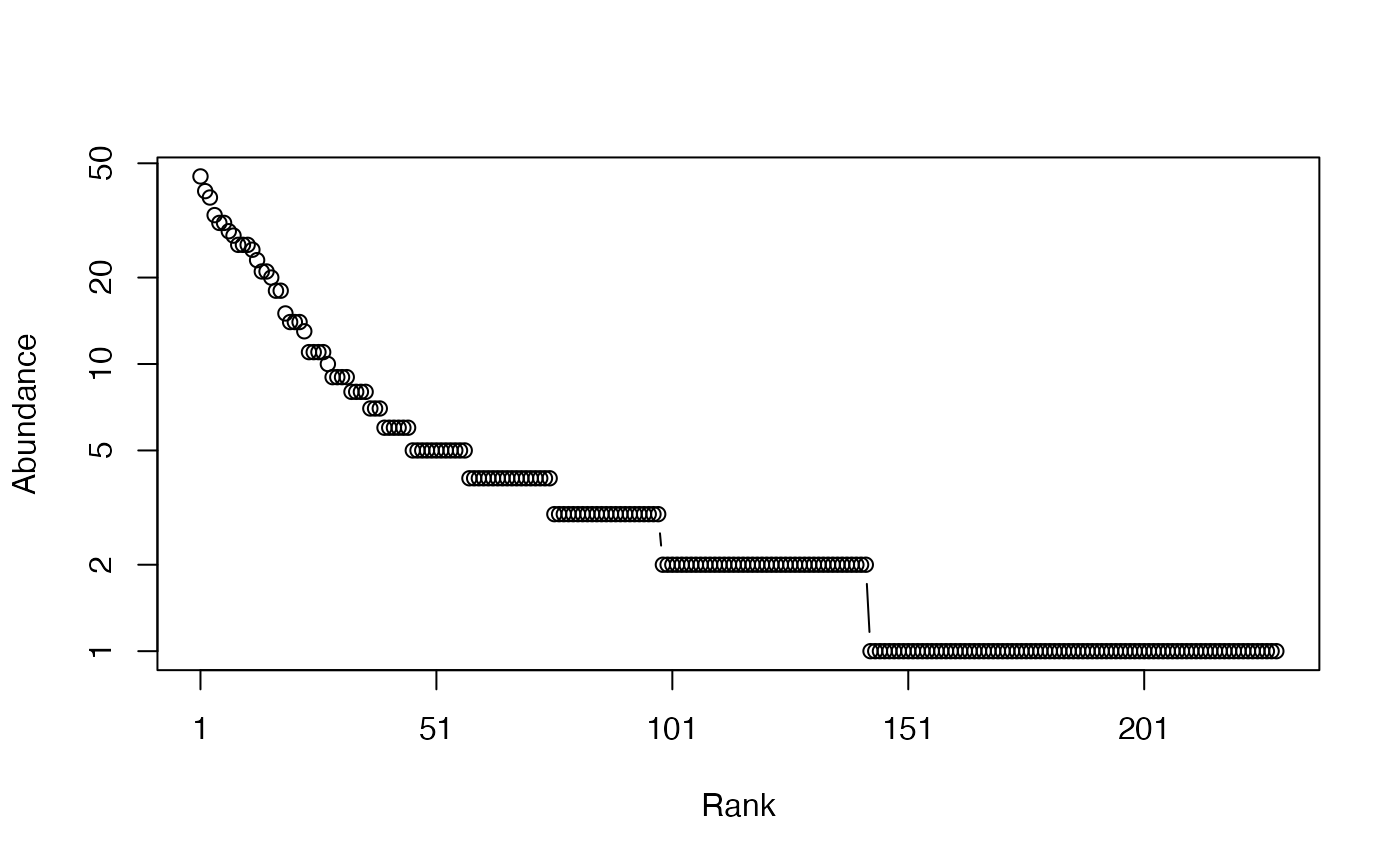Calculates the number of species from probability vector. The name is that of the estimator (the bias correction) used.

Richness(NorP, ...)
bcRichness(Ns, Correction = "Best", Alpha = 0.05, JackOver = FALSE, JackMax = 10,
CheckArguments = TRUE)
# S3 method for ProbaVector
Richness(NorP, ..., CheckArguments = TRUE, Ps = NULL)
# S3 method for AbdVector
Richness(NorP, Correction = "Best", Alpha = 0.05,
JackOver = FALSE, JackMax = 10,
Level = NULL, PCorrection = "Chao2015", Unveiling = "geom", RCorrection = "Rarefy",
..., CheckArguments = TRUE, Ns = NULL)
# S3 method for integer
Richness(NorP, Correction = "Best", Alpha = 0.05,
JackOver = FALSE, JackMax = 10,
Level = NULL, PCorrection = "Chao2015", Unveiling = "geom", RCorrection = "Rarefy",
..., CheckArguments = TRUE, Ns = NULL)
# S3 method for numeric
Richness(NorP, Correction = "Best", Alpha = 0.05,
JackOver = FALSE, JackMax = 10,
Level = NULL, PCorrection = "Chao2015", Unveiling = "geom", RCorrection = "Rarefy",
..., CheckArguments = TRUE, Ps = NULL, Ns = NULL)

## Arguments

Ps

A probability vector, summing to 1.

Ns

A numeric vector containing species abundances.

NorP

A numeric vector, an integer vector, an abundance vector (AbdVector) or a probability vector (ProbaVector). Contains either abundances or probabilities.

Correction

A string containing one of the possible corrections: "None" (no correction), "Jackknife", "iChao1", or "Chao1". "Best", the default value, is currently "Jackknife". Ignored by richness interpolation, and by extrapolation if PCorrection is not "None".

Alpha

The risk level, 5% by default, used to optimize the jackknife order.

JackOver

If TRUE, retain the jackknife order immediately superior to the optimal one, usually resulting in the overestimation of the number of species. Default is FALSE.

JackMax

The highest jackknife order allowed. Default is 10. Allowed values are between 1 and 9.

Level

The level of interpolation or extrapolation. It may be an a chosen sample size (an integer) or a sample coverage (a number between 0 and 1). Richness extrapolation require its asymptotic estimation depending on the choice of Correction.

PCorrection

A string containing one of the possible corrections to estimate a probability distribution in as.ProbaVector: "Chao2015" is the default value. If "None", the asymptotic distribution is not estimated and extrapolation relies only on the asymptotic estimator of richness. Used only for extrapolation.

Unveiling

A string containing one of the possible unveiling methods to estimate the probabilities of the unobserved species in as.ProbaVector: "geom" (the unobserved species distribution is geometric) is the default value. Used only for extrapolation.

RCorrection

A string containing a correction recognized by Richness to evaluate the total number of species in as.ProbaVector. "Rarefy" is the default value to estimate the number of species such that the entropy of the asymptotic distribution rarefied to the observed sample size equals the observed entropy of the data. Used only for extrapolation.

...

CheckArguments

Logical; if TRUE, the function arguments are verified. Should be set to FALSE to save time when the arguments have been checked elsewhere.

## Details

Bias correction requires the number of individuals. Use bcRichness and choose the Correction.

Chao correction techniques are from Chao (1984) and Chiu et al. (2015). The Jackknife estimator is calculated by a straight adaptation of the code by Ji-Ping Wang (jackknife in CRAN-archived package SPECIES). The optimal order is selected according to Burnham and Overton (1978; 1979). The argument JackOver allows selecting one order over the optimal one. Many other estimators are available elsewhere, the ones implemented here are necessary for other entropy estimations.

The functions are designed to be used as simply as possible. Richness is a generic method. If its first argument is an abundance vector, an integer vector or a numeric vector which does not sum to 1, the bias corrected function bcRichness is called.

Richness can be estimated at a specified level of interpolation or extrapolation, either a chosen sample size or sample coverage (Chao et al., 2014), rather than its asymptotic value. Extrapolation relies on the estimation of the asymptotic richness. If PCorrection is "None", then the asymptotic estimation of richness is made using the chosen Correction, else the asymtpotic distribution of the community is derived and its estimated richness adjusted so that the entropy of a sample of this distribution of the size of the actual sample has the entropy of the actual sample.

## Value

A named number equal to the estimated number of species. The name is the Correction, except for "SAC" (Species Accumulation Curve) for interpolation.

## Examples

# Load Paracou data (number of trees per species in two 1-ha plot of a tropical forest)
data(Paracou618)
# Ns is the total number of trees per species
Ns <- as.AbdVector(Paracou618.MC$Ns) # Species probabilities Ps <- as.ProbaVector(Paracou618.MC$Ns)
# Whittaker plot
plot(Ns)# Number of observed species
Richness(Ps)
#> None
#>  229
# Estimate the actual number of species
bcRichness(Ns, Correction = "Chao1")
#>    Chao1
#> 314.9348
bcRichness(Ns, Correction = "iChao1")
#>   iChao1
#> 333.7466
bcRichness(Ns, Correction = "Jackknife")
#> Jackknife 2
#>         359
bcRichness(Ns, Correction = "Jackknife", JackOver=TRUE)
#> Jackknife 3
#>         381